Like   Tweet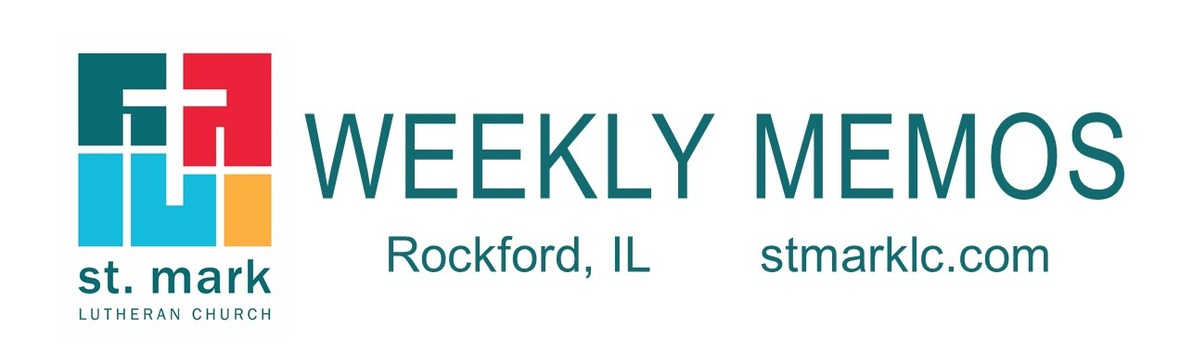table div table+table div table{width:100%;padding:0}table div table+table div table img{width:96.23%;padding:0;float:none}table div table+table div table td{width:100%;padding:0 1.88% 18px}/* styles */## Pastoral Reflection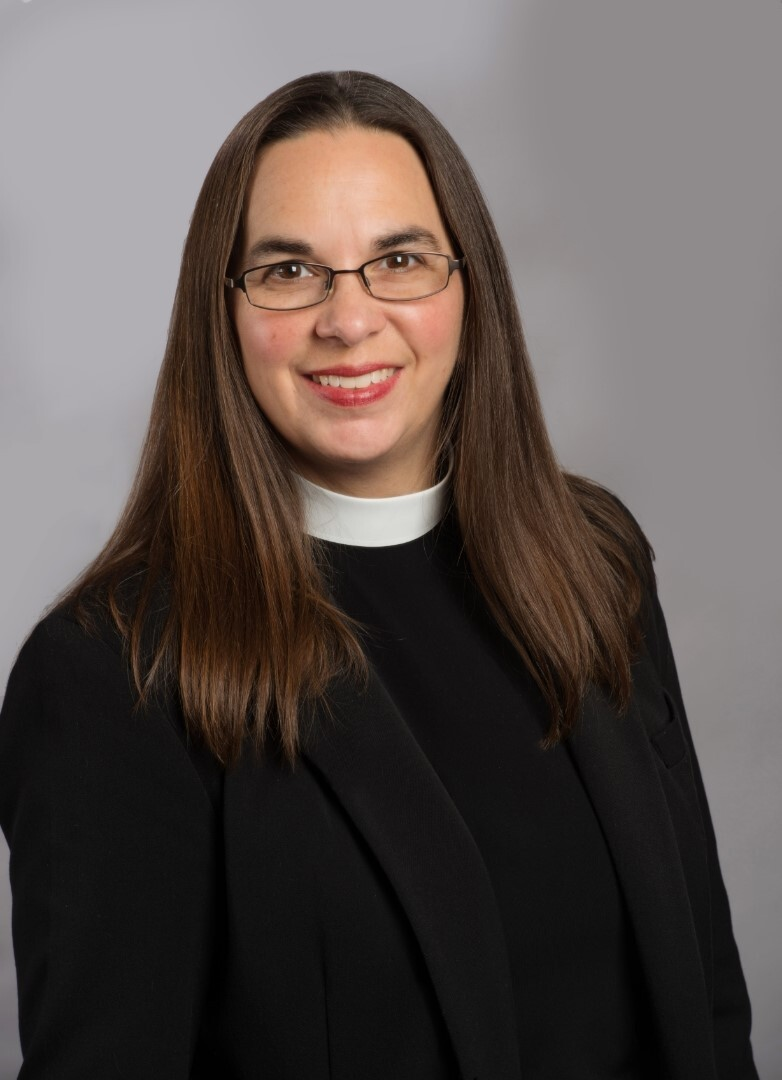The Joy of God's Creation!
Pastor Katrina Steingraeber

There is something about the sound of rain that is beautiful. And when I am privileged to wake up near a lake, that is just the best. I love being in God’s beautiful creation. Read more.

 table div table+table+table+table+table div table{width:100%;padding:0}table div table+table+table+table+table div table img{width:96.23%;padding:0;float:none}table div table+table+table+table+table div table td{width:100%;padding:0 1.88% 18px}/* styles */## This Week at St. Mark August 14 - 21, 2022

 table div table+table+table+table+table+table+table div table{width:100%;padding:0}table div table+table+table+table+table+table+table div table img{width:96.23%;padding:0;float:none}table div table+table+table+table+table+table+table div table td{width:100%;padding:0 1.88% 18px}/* styles */## Sunday, August 14 Back to School Blessing

9:00 A.M. Worship with Live-Stream
10:00 A.M. Fellowship Hour with coffee and donuts
11:00 A.M. Outdoor Worship on East Lawn

## Tuesday, August 16

10:00 A.M. Staff Meeting
10:30 A.M. St. Mark Duplicate Bridge
7:00 P.M. Executive Meeting

## Thursday, August 18

9:00 A.M. Cherry Valley Bridge Group
6:30 P.M. Blue Lights
6:30 P.M. Emotions Anonymous

## Friday, August 19

7:30 A.M. St. Mark Breakfast Group

## Saturday, August 20

9:00 A.M. Fellowship time for Erlene Martin
10:00 A.M. Celebration of Life for Erlene Martin

## Sunday, August 21

9:00 A.M. Worship with Live-Stream
10:00 A.M. Fellowship Hour with coffee and donuts
11:00 A.M. Outdoor Worship on East Lawn

 table div table+table+table+table+table+table+table+table+table div table{width:100%;padding:0}table div table+table+table+table+table+table+table+table+table div table img{width:96.23%;padding:0;float:none}table div table+table+table+table+table+table+table+table+table div table td{width:100%;padding:0 1.88% 18px}/* styles */## News and Events

 table div table+table+table+table+table+table+table+table+table+table+table div table{width:100%;padding:0}table div table+table+table+table+table+table+table+table+table+table+table div table img{width:96.23%;padding:0;float:none}table div table+table+table+table+table+table+table+table+table+table+table div table td{width:100%;padding:0 1.88% 18px}/* styles *//* styles */ August 14, 2022 - 10th Sunday after Pentecost 9:00 am - Traditional Worship in the Sanctuary (Prelude will begin at 8:55) 10:00 am - Fellowship hour with coffee and donuts 11:00 am - Praise Service in Fellowship Hall Our weekly live-stream will occur during the 9:00 am Sanctuary service. Follow this link for the live-stream service Sunday.
 table div table+table+table+table+table+table+table+table+table+table+table+table+table div table{width:100%;padding:0}table div table+table+table+table+table+table+table+table+table+table+table+table+table div table img{width:96.23%;padding:0;float:none}table div table+table+table+table+table+table+table+table+table+table+table+table+table div table td{width:100%;padding:0 1.88% 18px}/* styles */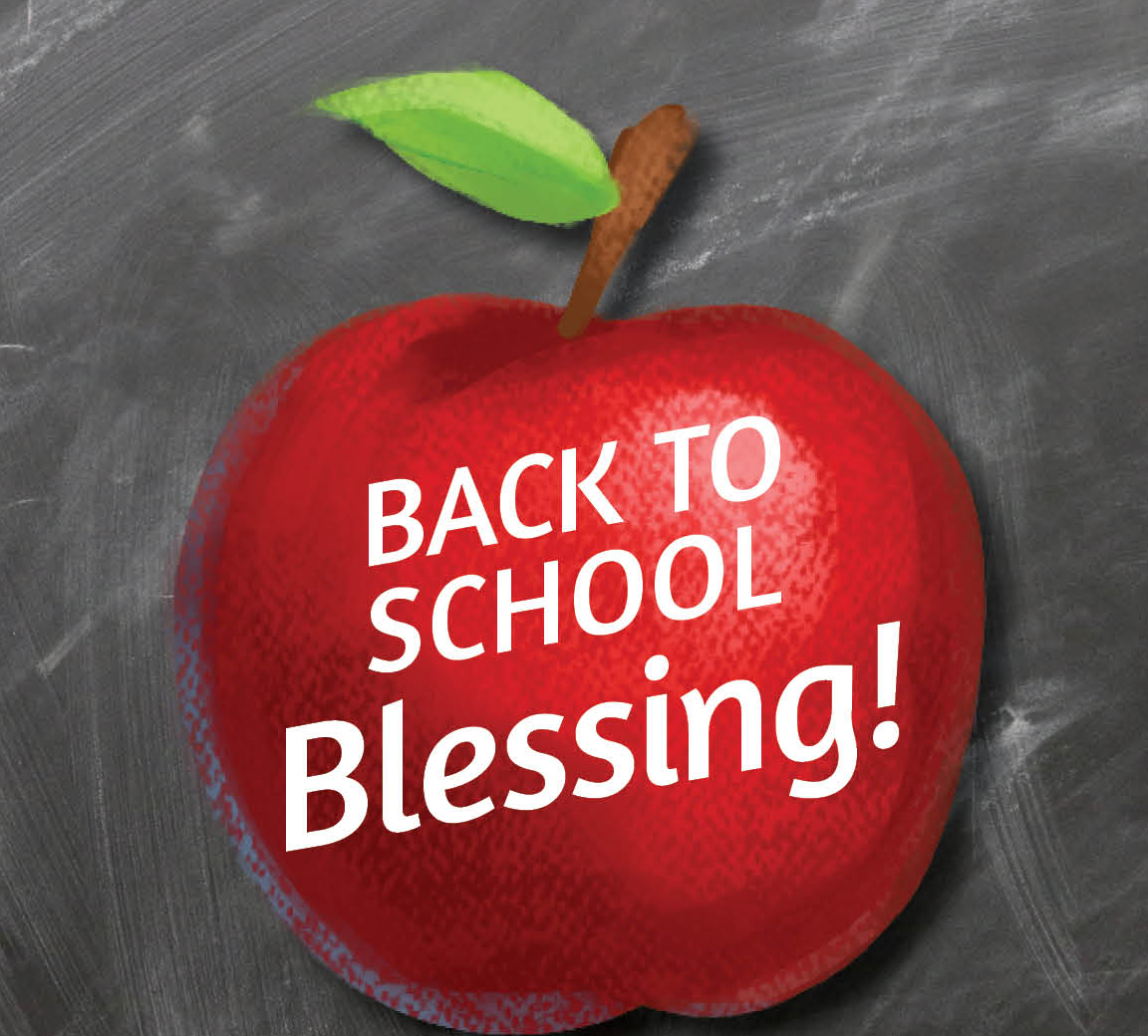Back to School Blessing
August 14 and 28, St. Mark will provide a back to school blessing for anyone returning to school during both services.

 table div table+table+table+table+table+table+table+table+table+table+table+table+table+table+table div table{width:100%;padding:0}table div table+table+table+table+table+table+table+table+table+table+table+table+table+table+table div table img{width:96.23%;padding:0;float:none}table div table+table+table+table+table+table+table+table+table+table+table+table+table+table+table div table td{width:100%;padding:0 1.88% 18px}/* styles */Welcome back to Sunday School!
Sunday school begins on September 18, and kids ages 3 through 6th grade are invited! We meet from 10:05 – 10:55 in the lower level. Each Sunday the whole group gathers for a welcome and brief introduction to the week’s Bible story. Then all students go to their classrooms for age-level activities.

New students will receive a Story Bible or NRSV Bible as a gift from the congregation. Please join us for the presentation of Bibles on September 18 at the 9:00 service!

 table div table+table+table+table+table+table+table+table+table+table+table+table+table+table+table+table+table div table{width:100%;padding:0}table div table+table+table+table+table+table+table+table+table+table+table+table+table+table+table+table+table div table img{width:96.23%;padding:0;float:none}table div table+table+table+table+table+table+table+table+table+table+table+table+table+table+table+table+table div table td{width:100%;padding:0 1.88% 18px}/* styles */Septemberfest - Sunday, September 11!
All are Welcome! We will have one worship service this morning at 10:30 a.m. outside under the tent. Worship, music, fellowship, food, and celebration!

There will be accessible seating for those who have mobility issues and plenty of ushers to help. Following the service there will be a catered lunch, the petting zoo will be back, and some activities.

We encourage you to invite friends and family to our weekend of celebration and opportunity.

 table div table+table+table+table+table+table+table+table+table+table+table+table+table+table+table+table+table+table+table div table{width:100%;padding:0}table div table+table+table+table+table+table+table+table+table+table+table+table+table+table+table+table+table+table+table div table img{width:96.23%;padding:0;float:none}table div table+table+table+table+table+table+table+table+table+table+table+table+table+table+table+table+table+table+table div table td{width:100%;padding:0 1.88% 18px}/* styles */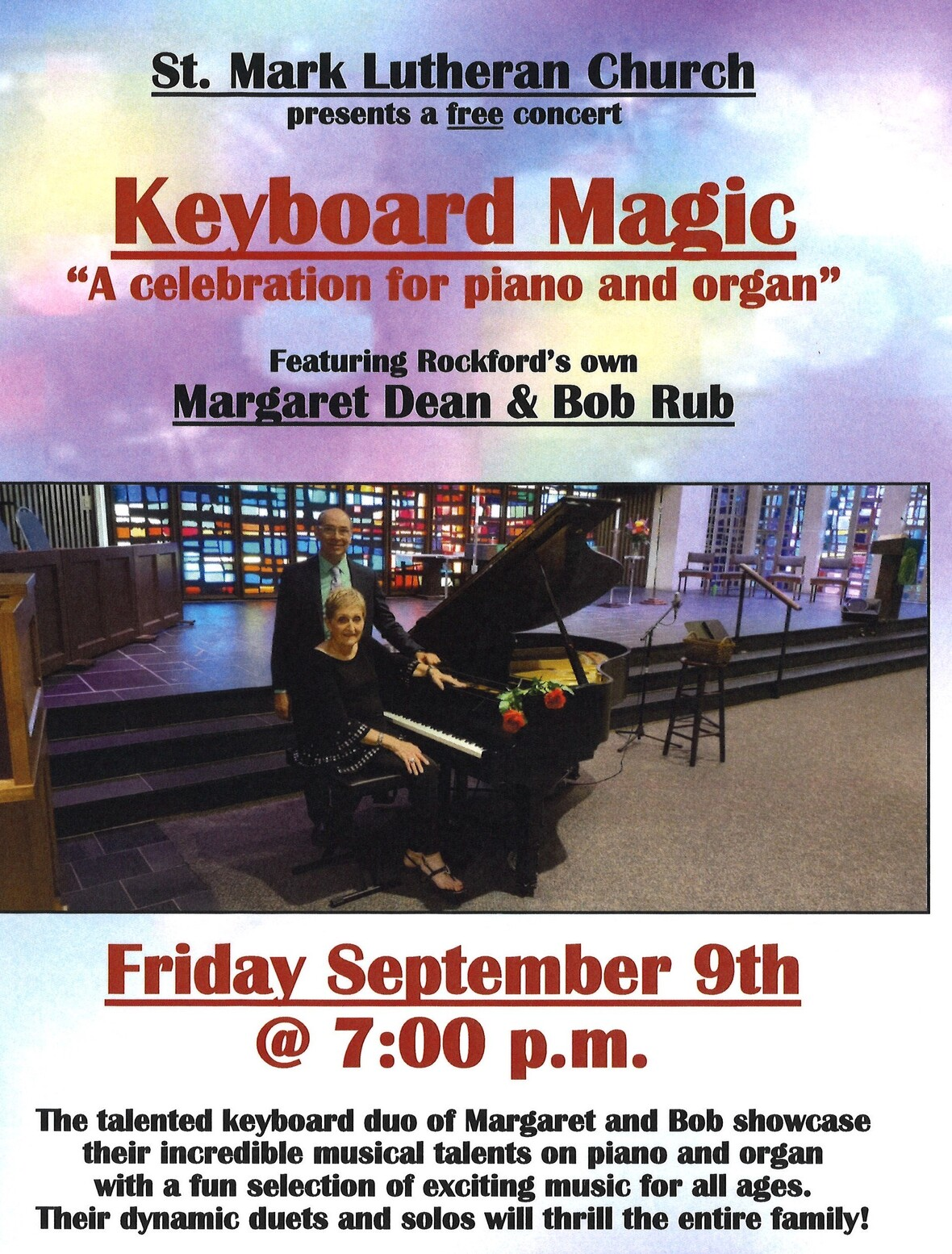table div table+table+table+table+table+table+table+table+table+table+table+table+table+table+table+table+table+table+table+table+table div table{width:100%;padding:0}table div table+table+table+table+table+table+table+table+table+table+table+table+table+table+table+table+table+table+table+table+table div table img{width:96.23%;padding:0;float:none}table div table+table+table+table+table+table+table+table+table+table+table+table+table+table+table+table+table+table+table+table+table div table td{width:100%;padding:0 1.88% 18px}/* styles */Lasagna Love - Meal Assembly Saturday, September 10th
We plan to make pans of lasagna. We are partnering with LasagnaLove.org.
Do you like to cook? Would you like to help assemble pans of lasagna? We will be feeding foster families in Rockford. Please sign-up in Fellowship Hall and say you will help, or call the church office. We need individuals, groups, families, friends, co-workers, etc.

Join us Saturday, September 10th at 10 AM, 11 AM, or 12 PM, shift at St. Mark. Wear your favorite apron!

 table div table+table+table+table+table+table+table+table+table+table+table+table+table+table+table+table+table+table+table+table+table+table+table div table{width:100%;padding:0}table div table+table+table+table+table+table+table+table+table+table+table+table+table+table+table+table+table+table+table+table+table+table+table div table img{width:96.23%;padding:0;float:none}table div table+table+table+table+table+table+table+table+table+table+table+table+table+table+table+table+table+table+table+table+table+table+table div table td{width:100%;padding:0 1.88% 18px}/* styles */Cheerful Giver Bookmark
Inside the ‘Friendship Binder’ in each row, you will find a “bookmark.” If you would like to be an online giver, you will find the QR code and a link to our online giving. If you are already an online giver and would like to actively place something in the offering plate, you are welcome to use the “bookmark.” The “bookmarks” will be available each Sunday in the binder for your use. Along with the bookmark, there are prayer request cards, offering envelopes and an attendance sheet. As you sign the attendance sheet pass the binder and take note of whom you are worshipping with. Thank you for supporting the ministries of St. Mark through both online and in-person giving. We could not do what we do without your support.

 table div table+table+table+table+table+table+table+table+table+table+table+table+table+table+table+table+table+table+table+table+table+table+table+table+table div table{width:100%;padding:0}table div table+table+table+table+table+table+table+table+table+table+table+table+table+table+table+table+table+table+table+table+table+table+table+table+table div table img{width:96.23%;padding:0;float:none}table div table+table+table+table+table+table+table+table+table+table+table+table+table+table+table+table+table+table+table+table+table+table+table+table+table div table td{width:100%;padding:0 1.88% 18px}/* styles */Women's Bible Study
The Women's Wednesday Morning Bible Study will resume on September 7th, 2022. We will meet at the Stockholm Inn for breakfast at 9:00 for good food and good fellowship. This is open to all women at St. Mark and their friends. This year we will begin with a 6-week study on Honoring Our Neighbor's Faith. We will discuss Buddhism, Hinduism, and Judaism. We will then begin a Bible Study on the book of Ecclesiastes from the Old Testament. If time permits we will finish the year studying the book of Romans. Our regularly scheduled Bible study will begin on September 28th. It is on this date that we will begin the study of Buddhism. The Bible Study is every Wednesday morning from 9:00-10:00 in the conference room. Please join us if you can as we study God's Word with discussion, sharing of life experiences, and prayer! If you have any questions call Deb Iverson (815-218-5152) or email at dsiverson5@gmail.com.

 table div table+table+table+table+table+table+table+table+table+table+table+table+table+table+table+table+table+table+table+table+table+table+table+table+table+table+table div table{width:100%;padding:0}table div table+table+table+table+table+table+table+table+table+table+table+table+table+table+table+table+table+table+table+table+table+table+table+table+table+table+table div table img{width:96.23%;padding:0;float:none}table div table+table+table+table+table+table+table+table+table+table+table+table+table+table+table+table+table+table+table+table+table+table+table+table+table+table+table div table td{width:100%;padding:0 1.88% 18px}/* styles */Nursery Volunteers Wanted!
For much of this summer, our intern Becca served as the nursery attendant during our 9:00 services. Now that she has headed back to college, we need some volunteers! We will move our 11:00 service back indoors beginning September 18, and so the nursery will also be open for both services after that date.

You can sign up here: Nursery Volunteer Sign-Up. Adults and youth are welcome, but youth under 16 need to serve in pairs.

Children are and always will be welcome in worship here at St. Mark, but we also recognize that nursery care can be a helpful option. There may be times when the nursery is open but no volunteer is available. In this case, you are still welcome to use the space to play or relax with your child. We are always looking for ways to better support our members and visitors. If you have questions or want more information, please email julie@stmarklc.com.

 table div table+table+table+table+table+table+table+table+table+table+table+table+table+table+table+table+table+table+table+table+table+table+table+table+table+table+table+table+table div table{width:100%;padding:0}table div table+table+table+table+table+table+table+table+table+table+table+table+table+table+table+table+table+table+table+table+table+table+table+table+table+table+table+table+table div table img{width:96.23%;padding:0;float:none}table div table+table+table+table+table+table+table+table+table+table+table+table+table+table+table+table+table+table+table+table+table+table+table+table+table+table+table+table+table div table td{width:100%;padding:0 1.88% 18px}/* styles *//* styles */ The youth recently volunteered and had a tour at “Miss Carly’s.” Our youth listened and learned about Miss Carly’s. One of our youth heard that they could use old tablets. Have you got old “tablets” to recycle? Please drop them off by August 28th at the church office. We will get them to Miss Carly’s. Please e-mail Melinda Alekna if you have questions. melinda@stmarklc.com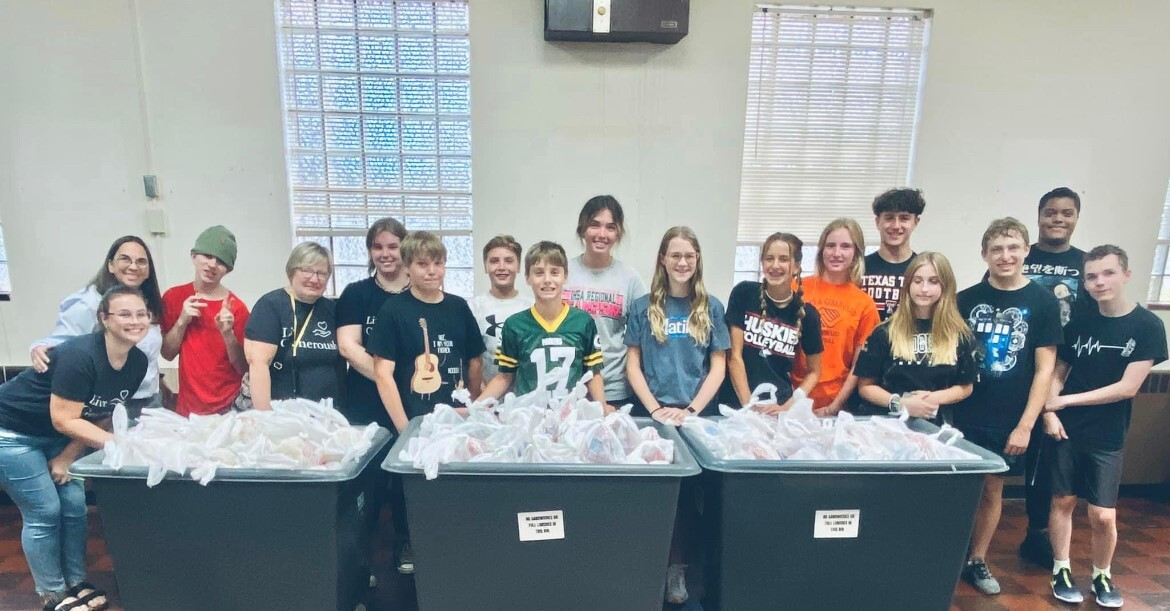table div table+table+table+table+table+table+table+table+table+table+table+table+table+table+table+table+table+table+table+table+table+table+table+table+table+table+table+table+table+table+table+table div table{width:100%;padding:0}table div table+table+table+table+table+table+table+table+table+table+table+table+table+table+table+table+table+table+table+table+table+table+table+table+table+table+table+table+table+table+table+table div table img{width:96.23%;padding:0;float:none}table div table+table+table+table+table+table+table+table+table+table+table+table+table+table+table+table+table+table+table+table+table+table+table+table+table+table+table+table+table+table+table+table div table td{width:100%;padding:0 1.88% 18px}/* styles */The Sew & Sew group is looking for new members.
We meet on the second and fourth Thursdays of each month from 12:30 to 3:00 PM. Don’t be scared away by the fact that we sew and make quilts. You don’t have to be able to sew because there are several steps in putting together quilts or port pillows that do not require sewing. Some of us take fabric home and work on quilt tops at home and then bring it back to church to finish the quilt with the batting and backing. The best part is our super machine quilter Chris Allen takes a pinned quilt home and brings it back all beautifully quilted. Then we have members that like to do hand stitching, so they sit and hand sew the binding to the back of the quilt along with our labels that read, "Made with love by St. Mark Lutheran Sew and Sews".

We make quilts for each member of the families that move into Habitat for Humanity homes, and quilts for foster children. We make soft fleece throws for the children at Rock House Kids, and port pillows for cancer patients to use on their car seat belts.
These warm quilts and blankets and the port pillows are so appreciated by each recipient, please come and join us. For questions: please contact Nancy Eckburg at 815-988-2211 fancynanburg@comcast.net or Nancy Leonard at 815-978-1406 nan973@yahoo.com.

 table div table+table+table+table+table+table+table+table+table+table+table+table+table+table+table+table+table+table+table+table+table+table+table+table+table+table+table+table+table+table+table+table+table+table div table{width:100%;padding:0}table div table+table+table+table+table+table+table+table+table+table+table+table+table+table+table+table+table+table+table+table+table+table+table+table+table+table+table+table+table+table+table+table+table+table div table img{width:96.23%;padding:0;float:none}table div table+table+table+table+table+table+table+table+table+table+table+table+table+table+table+table+table+table+table+table+table+table+table+table+table+table+table+table+table+table+table+table+table+table div table td{width:100%;padding:0 1.88% 18px}/* styles */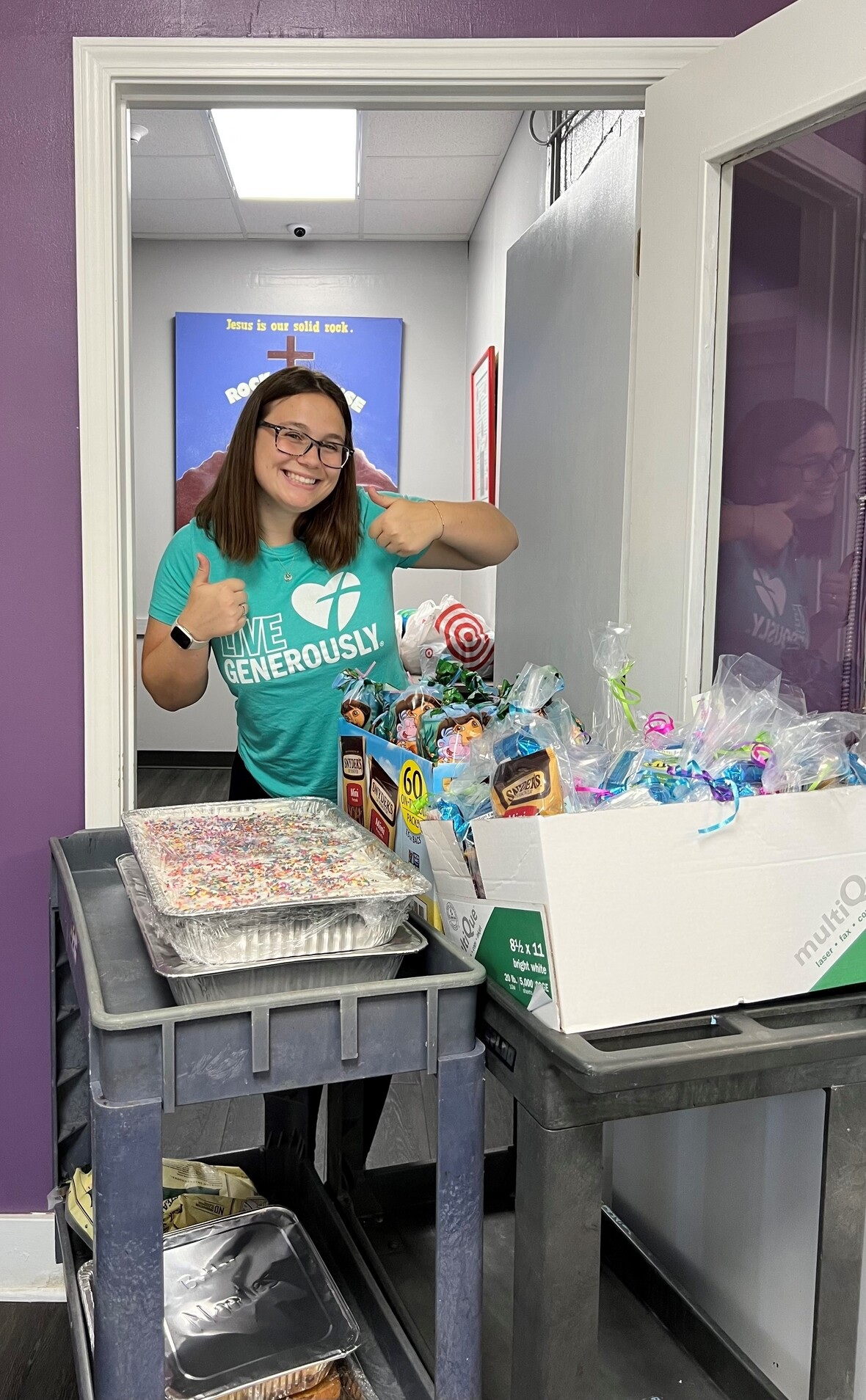Sign-up to make a meal for Rock House kids.
The dates are September 21 and October 19 which we need commitments for. Ask your friends, Co-workers, and family to help. We have ideas and recipes! E-mail or call Melinda Alekna 815-871-0390,melinda@stmarklc.com.

 table div table+table+table+table+table+table+table+table+table+table+table+table+table+table+table+table+table+table+table+table+table+table+table+table+table+table+table+table+table+table+table+table+table+table+table+table div table{width:100%;padding:0}table div table+table+table+table+table+table+table+table+table+table+table+table+table+table+table+table+table+table+table+table+table+table+table+table+table+table+table+table+table+table+table+table+table+table+table+table div table img{width:96.23%;padding:0;float:none}table div table+table+table+table+table+table+table+table+table+table+table+table+table+table+table+table+table+table+table+table+table+table+table+table+table+table+table+table+table+table+table+table+table+table+table+table div table td{width:100%;padding:0 1.88% 18px}/* styles */## Youth Ministrytable div table+table+table+table+table+table+table+table+table+table+table+table+table+table+table+table+table+table+table+table+table+table+table+table+table+table+table+table+table+table+table+table+table+table+table+table+table+table+table div table{width:100%;padding:0}table div table+table+table+table+table+table+table+table+table+table+table+table+table+table+table+table+table+table+table+table+table+table+table+table+table+table+table+table+table+table+table+table+table+table+table+table+table+table+table div table img{width:96.23%;padding:0;float:none}table div table+table+table+table+table+table+table+table+table+table+table+table+table+table+table+table+table+table+table+table+table+table+table+table+table+table+table+table+table+table+table+table+table+table+table+table+table+table+table div table td{width:100%;padding:0 1.88% 18px}/* styles */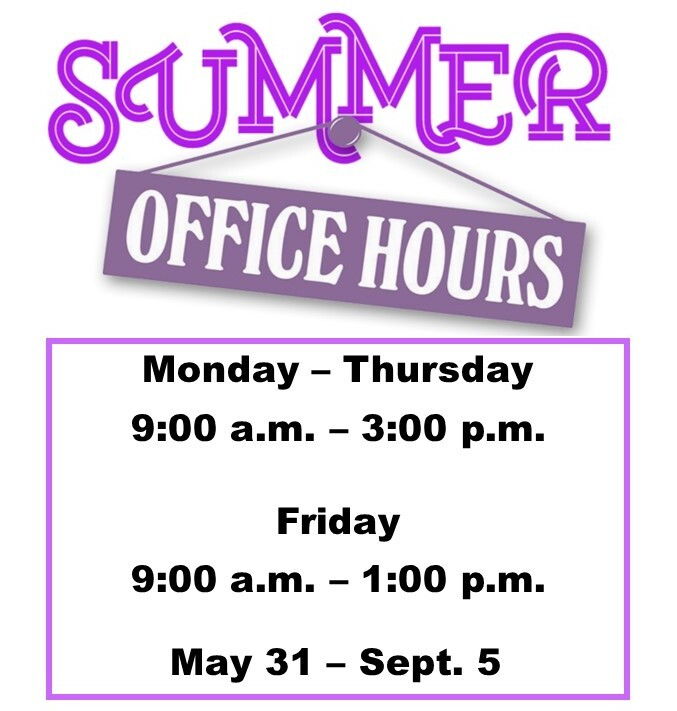table div table+table+table+table+table+table+table+table+table+table+table+table+table+table+table+table+table+table+table+table+table+table+table+table+table+table+table+table+table+table+table+table+table+table+table+table+table+table+table+table+table div table{width:100%;padding:0}table div table+table+table+table+table+table+table+table+table+table+table+table+table+table+table+table+table+table+table+table+table+table+table+table+table+table+table+table+table+table+table+table+table+table+table+table+table+table+table+table+table div table img{width:96.23%;padding:0;float:none}table div table+table+table+table+table+table+table+table+table+table+table+table+table+table+table+table+table+table+table+table+table+table+table+table+table+table+table+table+table+table+table+table+table+table+table+table+table+table+table+table+table div table td{width:100%;padding:0 1.88% 18px}/* styles */## Our St. Mark members:

Jim Tooley, Carol Kilts, Marsha Carlson, Dennis Pitcock, Linda Vivian, Tim Panjkovich, Rita Olson, Fritzi Panjkovich, Bev Johnson, Jack Sowl, Lorine Kortus, Jan Cotter, Diane Swanson, Sherri Lindquist, Bette Patterson, Keith Haggestad.

## Family and friends of St. Mark members:

Becky Belk, Janet Webster, Brock Lawrence, Carrie Nelson, Bill Hart, Roger Clark, Ellie Krepfle, Carl Steingraeber, Don Minnis, Tom Montgomery, Jon Baylander, Debbie Park, John Mortensen, Carolyn Neagle, Sue Flott, Bob Pollack, Jenny Hobby, Bill King, Clyde Webster, Conrad Semmelroth, Nici King, Debbie Adams, Linda Johnson, Eva Love Sherbondy, Norma Comstock, Carolyn King.

## Those serving in the military and their families:

Logan Gehrke, Hunter Haggestad, Tristan Davenport, Scot Hornick,
Bailey Panjkovich, Jim Tammen, Brandon Ballenger, Taylor Eversole.

Strengthen and encourage those in public health services and in the medical profession: care-givers, nurses, attendants, doctors, all who commit themselves to care for the sick and their families.

 table div table+table+table+table+table+table+table+table+table+table+table+table+table+table+table+table+table+table+table+table+table+table+table+table+table+table+table+table+table+table+table+table+table+table+table+table+table+table+table+table+table+table+table+table div table{width:100%;padding:0}table div table+table+table+table+table+table+table+table+table+table+table+table+table+table+table+table+table+table+table+table+table+table+table+table+table+table+table+table+table+table+table+table+table+table+table+table+table+table+table+table+table+table+table+table div table img{width:96.23%;padding:0;float:none}table div table+table+table+table+table+table+table+table+table+table+table+table+table+table+table+table+table+table+table+table+table+table+table+table+table+table+table+table+table+table+table+table+table+table+table+table+table+table+table+table+table+table+table+table div table td{width:100%;padding:0 1.88% 18px}/* styles *//* styles */ Summer Office Hours Monday through Thursday 9:00 A.M. - 3:00 P.M. Friday - 9:00 A.M. - 1:00 P.M. Telephone: 815-398-3557 Publications St. Mark Memos, the weekly e-newsletter, is distributed each Thursday. The deadline for submission is Monday by 3:00 P.M. Please click to send submissions. Worship Services Miss a Sunday at St. Mark? Please visit our website and watch the service online. Please click to watch.
 table div table+table+table+table+table+table+table+table+table+table+table+table+table+table+table+table+table+table+table+table+table+table+table+table+table+table+table+table+table+table+table+table+table+table+table+table+table+table+table+table+table+table+table+table+table+table div table{width:100%;padding:0}table div table+table+table+table+table+table+table+table+table+table+table+table+table+table+table+table+table+table+table+table+table+table+table+table+table+table+table+table+table+table+table+table+table+table+table+table+table+table+table+table+table+table+table+table+table+table div table img{width:96.23%;padding:0;float:none}table div table+table+table+table+table+table+table+table+table+table+table+table+table+table+table+table+table+table+table+table+table+table+table+table+table+table+table+table+table+table+table+table+table+table+table+table+table+table+table+table+table+table+table+table+table+table div table td{width:100%;padding:0 1.88% 18px}/* styles */